Latest Teaching jobs   »   Study Notes on Important Facts and...

# Study Notes on Important Facts and Formulae- Math Study Notes for CTET Exam

``

## Study Notes on Important Facts and Formulae

Mathematics is an equally important section for CTET, MPTET, KVS & DSSSB Exams and has even more abundant importance in some other exams conducted by central or state govt. Generally, there are questions asked related to basic concepts, Facts and Formulae of the Arithmetic.

To let you make the most of Mathematics section, we are providing important facts related to the Number System, HCF, LCM and Decimals. At least 5-6 questions are asked from these topics in most of the teaching exams. We wish you all the best of luck to come over the fear of the Mathematics section.

How to Overcome Exam Fever, Especially When You Fear Maths

• FACTORS AND MULTIPLES: If a number ‘a’ divides another number ‘b’ exactly, then ‘a’ is a factor of ‘b’. In this case, ‘b’ is called a multiple of ‘a’.

Ex. Find the total number of factors of 240.
Sol. 240 = 2×2×2×2×3×5
= 24 × 31 × 51
total factors = 4+1 × 1+1 × 1+1=20

• HCF (Highest common Factor): The HCF of two or more than two numbers is the greatest number that divides each of them exactly.

Ex. HCF of 36, 72, 108
Sol. 36 = 22 × 33
72 = 23 × 32
108 = 22 × 33
HCF = 22 × 32 = 36
Practice Mathematics Quiz Here for DSSSB Exam

• LCM (Lowest Common Multiple): The least number which is exactly divisible by each one of the given numbers is called their LCM

Ex. LCM of 87 and 145
Sol. 87= 3×29
145 = 5×29
LCM= 3×5×29= 435

• Product of two numbers = Products of their HCF and LCM and LCM is always divisible by HCF.

Ex. The sum and difference of the LCM and HCF of two numbers are 112 and 72 respectively. If the one of the numbers is 46, find the 2nd number.

Sol.
L+H = 112
L-H = 72
___________
2H = 40
___________
H= 20
L+20 = 112
L= 92
1st number = 46
Let the 2nd No. = x
46×x = 92×20
x= 40

• HCF and LCM of fractions: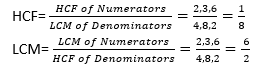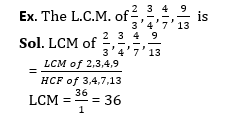• HCF of two numbers = HCF of sum of the numbers and their LCM.
• Decimal fraction: Fraction in which denominators are power of 10 are known as decimal fractions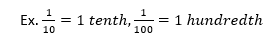Mathematics Quiz For CTET Exam : Attempt Daily Quizzes

• Recurring Decimal: If in a decimal fraction, a figure or a set of figures is repeated continuously, then such a number is called a recurring decimal.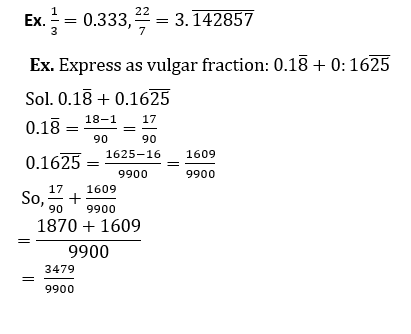• Basic formulae:
• (a+b) (a-b) = (a²-b²)
• (a-b)² = a²+b²-2ab
• (a+b)²= a²+b²+2ab

Ex. (x+y)=21 and xy=108, then what is the value of x²+y²
Sol. Sol. x+y= 21
xy= 108
(x+y)² = x²+y²+2xy
(21)² = x²+y²+2×108
441 = x²+y²+216
x²+y² = 225
Mathematics Study Notes For All Teaching Exams

• (a+b+c)² = a²+b²+c²+2(ab+bc+ca)
• (a³+b³)= (a+b) (a²+b²-ab)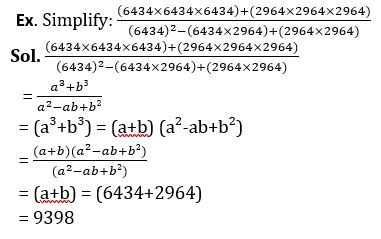• (a³-b³)= (a-b) (a²+b²+ab)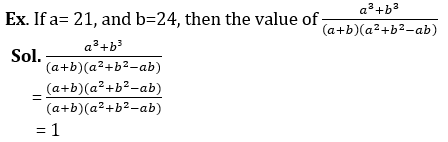• (a³+b³c³-3abc)= (a+b+c) (a²+b²+c²-ab-bc-ca)
• When a+b+c = 0, then a³+b³+c³= 3abc
• (a+b)²+(a-b)²= 2(a²+b²)
• (a-b)³ = a³-b³-3ab(a-b)
• (a+b)²-(a-b)² = 4ab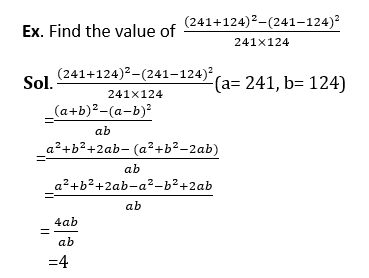• BODMAS Rule: The rule depicts the correct sequence in which operations are to be executed, so as to find out the value of given expression.

Ex. ‘B’ for Bracket, ‘O’ for of, ‘D’ for Division, ‘M’ for Multiplication, ‘A’ or Addition and ‘S’ for Subtraction.
Ex.  The value of (6.5×7.25+8.5×19.5+4.5-8.5)
Sol. (6.5×7.25+8.5×19.5+4.5-8.5)
= 47.125+165.75+4.5-8.5
= 217.375-8.5
= 208.875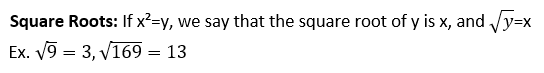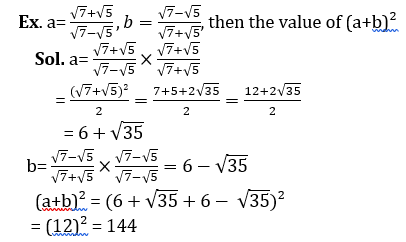Practice More Mathematics  Quiz Here

Check More Mathematics Study Notes Here

Sharing is caring!

Thank You, Your details have been submitted we will get back to you.

### TOPICS:

•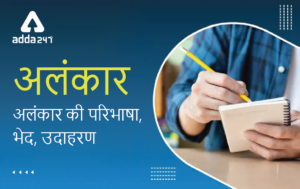अलंकार - परिभाष�...
•विशेषण- परिभाष�...
•लेखन कौशल की पर�...
•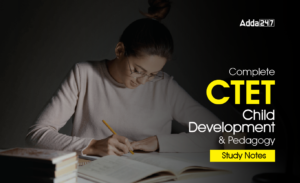Complete CTET Child Development and Peda...
•New Education Policy(NEP) PDF - नई �...
•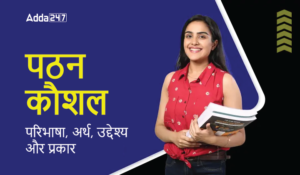पठन कौशल- परिभा�...